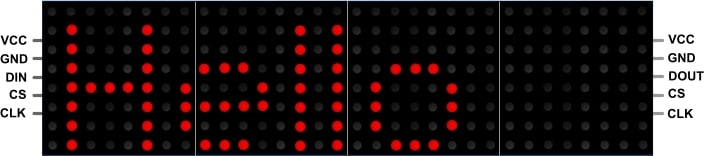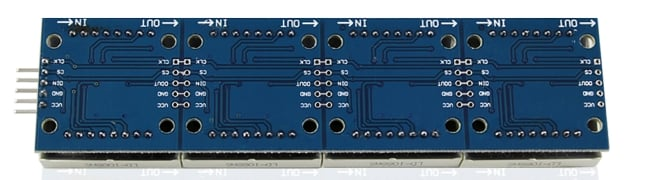# MAX7219 4-in-1 Dot Matrix Display Module (Green)

DL-025
12 In stock
R 132.25
When you need some help driving a lot of LEDs, the MAX7219 is your best friend. The annoying thing about multiplexing is that you need to constantly refresh the display to keep the image stable. The Max7219 does all the multiplexing for you. This nice breakout use four 8x8 Dot...
R 132.25
Terms & conditions

When you need some help driving a lot of LEDs, the MAX7219 is your best friend. The annoying thing about multiplexing is that you need to constantly refresh the display to keep the image stable. The Max7219 does all the multiplexing for you. This nice breakout use four 8x8 Dot Matrix displays which gives you a 32 x 8 Dot matrix display.

### Pinout### Back of the Display### Example Sketch:

• Required Libraries:
``````#include <SPI.h>
#include <Max72xxPanel.h>

int pinCS = 10; // Attach CS to this pin, DIN to MOSI and CLK to SCK
int numberOfHorizontalDisplays = 1;
int numberOfVerticalDisplays = 4;

Max72xxPanel matrix = Max72xxPanel(pinCS, numberOfHorizontalDisplays, numberOfVerticalDisplays);

String tape = "HELLO WORLD";
int wait = 100; // In milliseconds

int spacer = 1;
int width = 5 + spacer; // The font width is 5 pixels

void setup() {

matrix.setIntensity(15); // Use a value between 0 and 15 for brightness

//  matrix.setPosition(0, 0, 0); // The first display is at <0, 0>
//  matrix.setPosition(1, 1, 0); // The second display is at <1, 0>
//  matrix.setPosition(2, 2, 0); // The third display is at <2, 0>
//  matrix.setPosition(3, 3, 0); // And the last display is at <3, 0>
//  ...
//  matrix.setRotation(0, 2);    // The first display is position upside down
matrix.setRotation(3);    // The same hold for the last display
}

void loop() {

for ( int i = 0 ; i < width * tape.length() + matrix.width() - 1 - spacer; i++ ) {

matrix.fillScreen(LOW);

int letter = i / width;
int x = (matrix.width() - 1) - i % width;
int y = (matrix.height() - 8) / 2; // center the text vertically

while ( x + width - spacer >= 0 && letter >= 0 ) {
if ( letter < tape.length() ) {
matrix.drawChar(x, y, tape[letter], HIGH, LOW, 1);
}

letter--;
x -= width;
}

matrix.write(); // Send bitmap to display

delay(wait);
}

}``````
Write a review